Principal Component Analysis PCA Learning Representations Dimensionality Reduction

• Slides: 22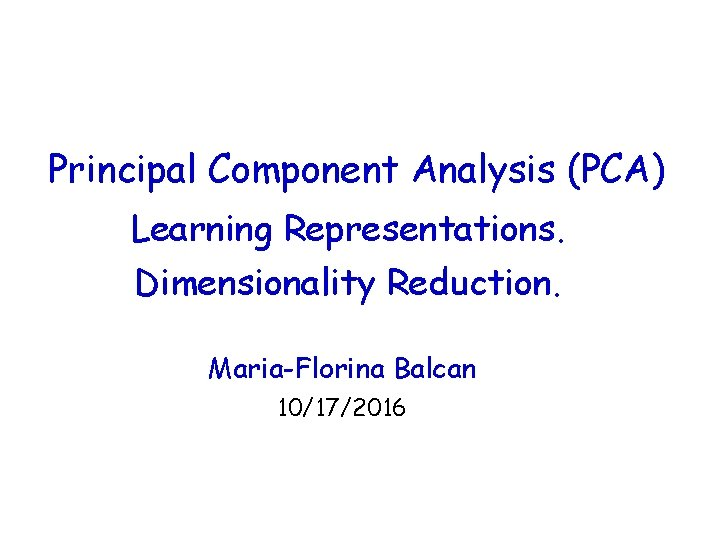Principal Component Analysis (PCA) Learning Representations. Dimensionality Reduction. Maria-Florina Balcan 10/17/2016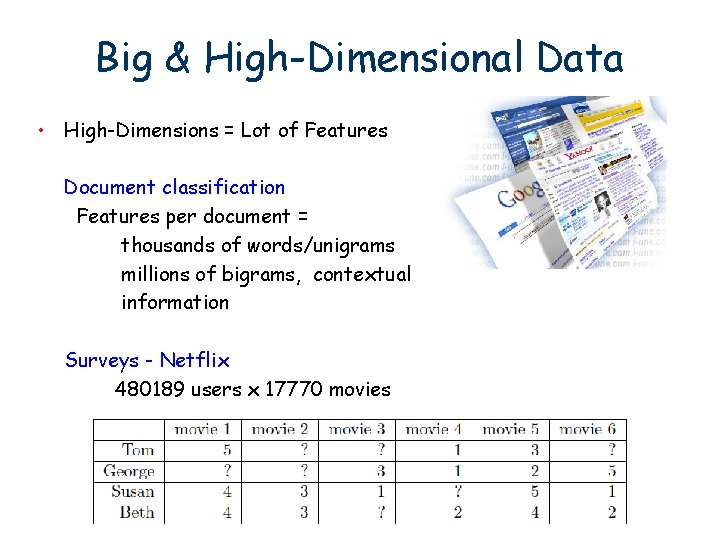Big & High-Dimensional Data • High-Dimensions = Lot of Features Document classification Features per document = thousands of words/unigrams millions of bigrams, contextual information Surveys - Netflix 480189 users x 17770 moviesBig & High-Dimensional Data • High-Dimensions = Lot of Features MEG Brain Imaging 120 locations x 500 time points x 20 objects Or any high-dimensional image data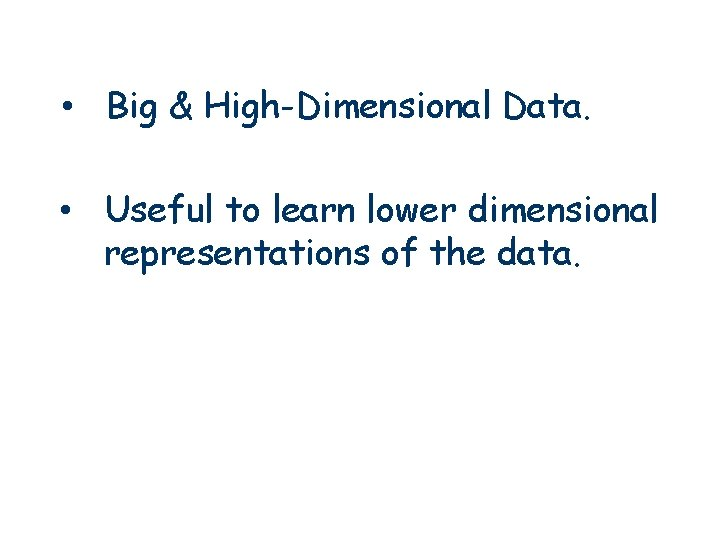• Big & High-Dimensional Data. • Useful to learn lower dimensional representations of the data.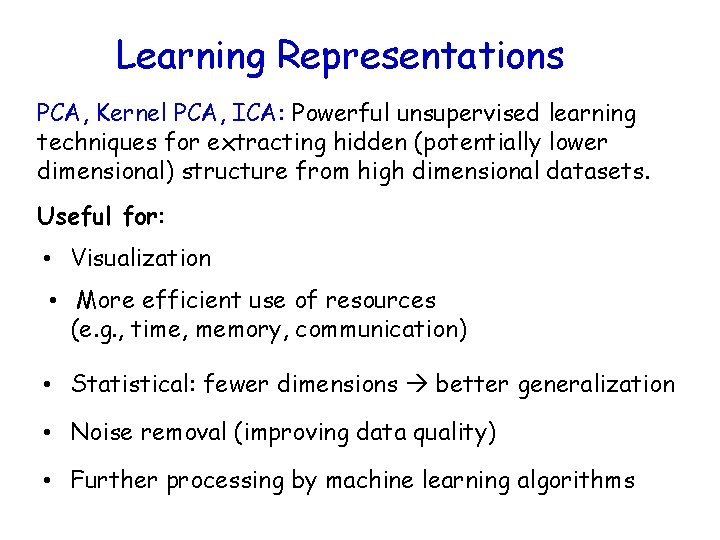Learning Representations PCA, Kernel PCA, ICA: Powerful unsupervised learning techniques for extracting hidden (potentially lower dimensional) structure from high dimensional datasets. Useful for: • Visualization • More efficient use of resources (e. g. , time, memory, communication) • Statistical: fewer dimensions better generalization • Noise removal (improving data quality) • Further processing by machine learning algorithmsPrincipal Component Analysis (PCA) What is PCA: Unsupervised technique for extracting variance structure from high dimensional datasets. • PCA is an orthogonal projection or transformation of the data into a (possibly lower dimensional) subspace so that the variance of the projected data is maximized.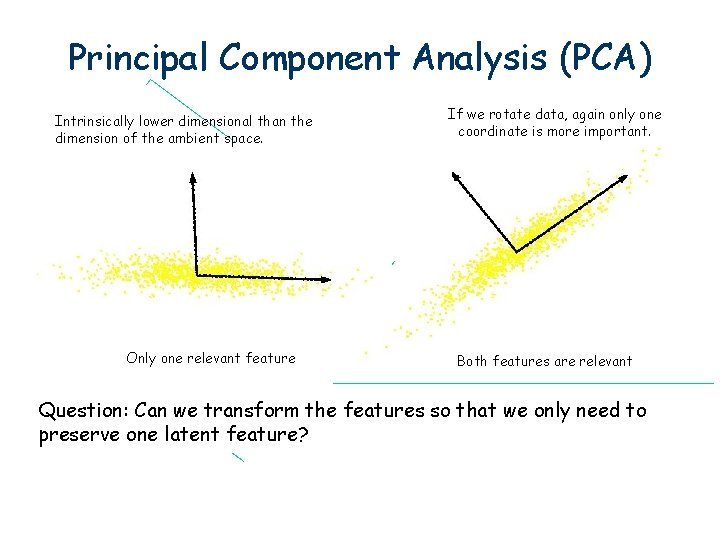Principal Component Analysis (PCA) Intrinsically lower dimensional than the dimension of the ambient space. Only one relevant feature If we rotate data, again only one coordinate is more important. Both features are relevant Question: Can we transform the features so that we only need to preserve one latent feature?Principal Component Analysis (PCA) In case where data lies on or near a low d-dimensional linear subspace, axes of this subspace are an effective representation of the data. Identifying the axes is known as Principal Components Analysis, and can be obtained by using classic matrix computation tools (Eigen or Singular Value Decomposition).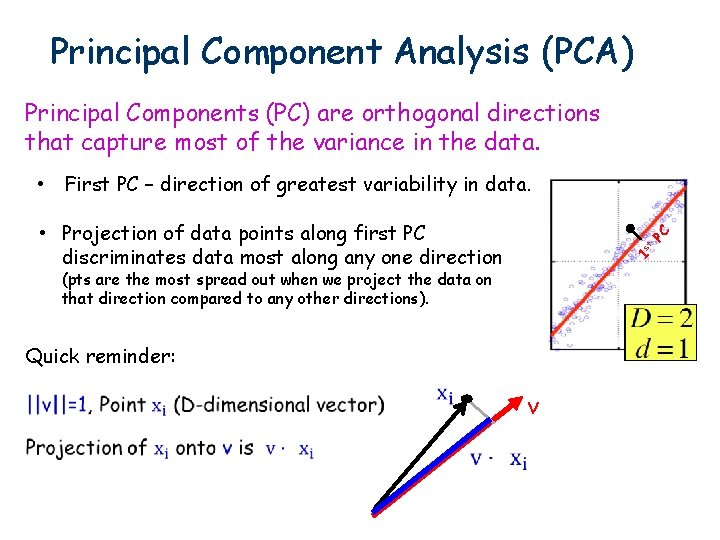Principal Component Analysis (PCA) Principal Components (PC) are orthogonal directions that capture most of the variance in the data. • First PC – direction of greatest variability in data. 1 st PC • Projection of data points along first PC discriminates data most along any one direction (pts are the most spread out when we project the data on that direction compared to any other directions). Quick reminder: vPrincipal Component Analysis (PCA) • 1 st PC – direction of greatest variability in data. PC 1 st 2 nd PC Principal Components (PC) are orthogonal directions that capture most of the variance in the data. • 2 nd PC – Next orthogonal (uncorrelated) direction of greatest variability (remove all variability in first direction, then find next direction of greatest variability) • And so on …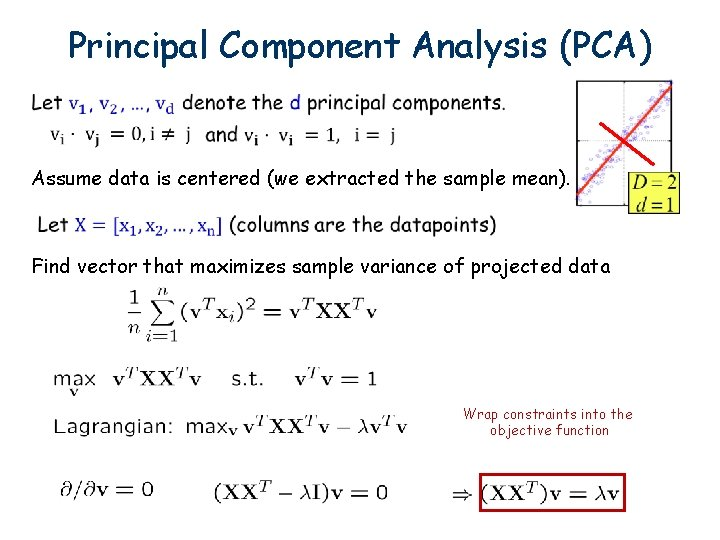Principal Component Analysis (PCA) Assume data is centered (we extracted the sample mean). Find vector that maximizes sample variance of projected data Wrap constraints into the objective function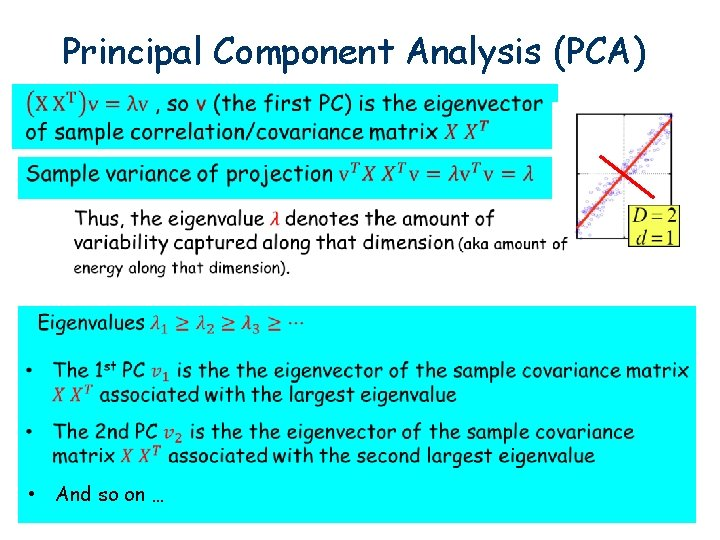Principal Component Analysis (PCA) • And so on …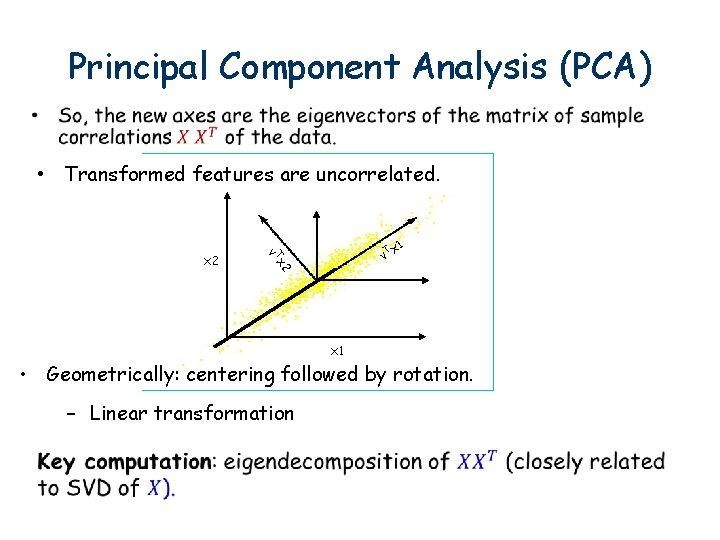Principal Component Analysis (PCA) • Transformed features are uncorrelated. Tx v 1 2 x 2 Tx v x 1 • Geometrically: centering followed by rotation. – Linear transformation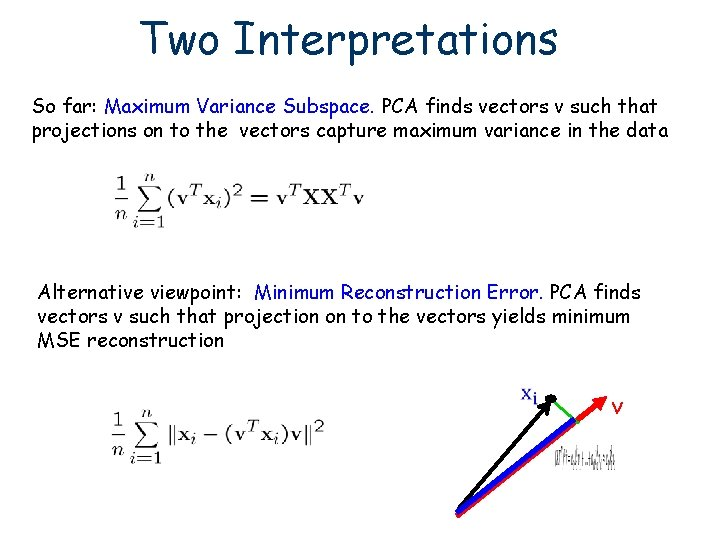Two Interpretations So far: Maximum Variance Subspace. PCA finds vectors v such that projections on to the vectors capture maximum variance in the data Alternative viewpoint: Minimum Reconstruction Error. PCA finds vectors v such that projection on to the vectors yields minimum MSE reconstruction v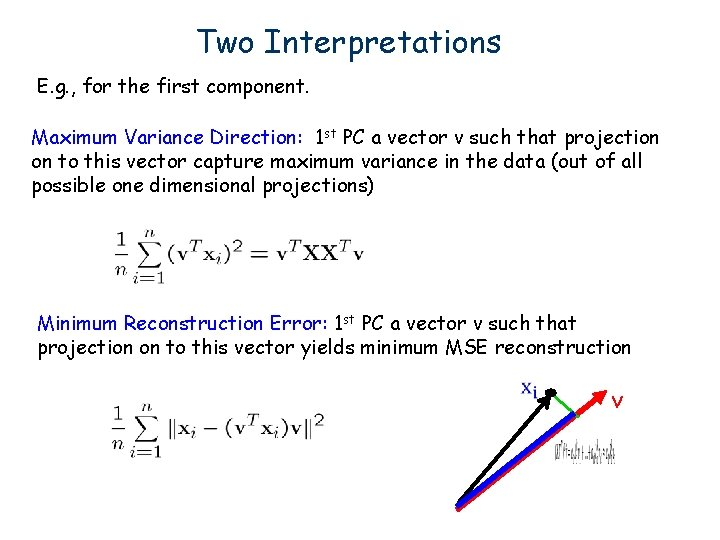Two Interpretations E. g. , for the first component. Maximum Variance Direction: 1 st PC a vector v such that projection on to this vector capture maximum variance in the data (out of all possible one dimensional projections) Minimum Reconstruction Error: 1 st PC a vector v such that projection on to this vector yields minimum MSE reconstruction vWhy? Pythagorean Theorem E. g. , for the first component. Maximum Variance Direction: 1 st PC a vector v such that projection on to this vector capture maximum variance in the data (out of all possible one dimensional projections) Minimum Reconstruction Error: 1 st PC a vector v such that projection on to this vector yields minimum MSE reconstruction blue 2 + green 2 = black 2 is fixed (it’s just the data) So, maximizing blue 2 is equivalent to minimizing green 2 v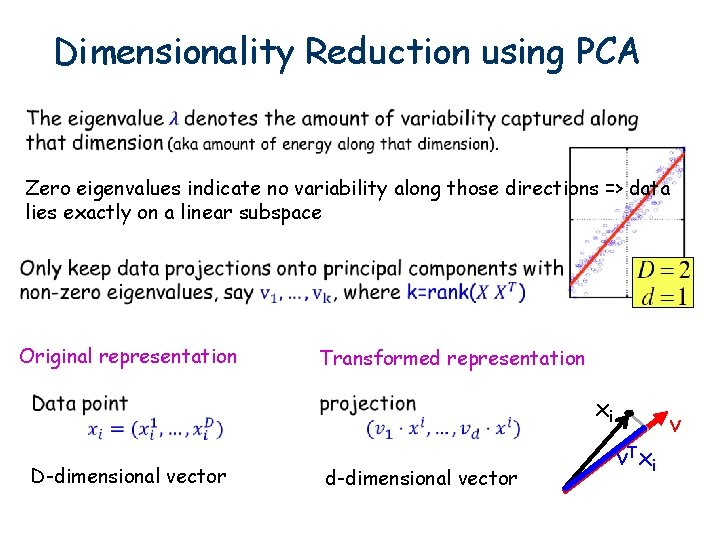Dimensionality Reduction using PCA Zero eigenvalues indicate no variability along those directions => data lies exactly on a linear subspace Original representation Transformed representation xi D-dimensional vector d-dimensional vector v. T xi v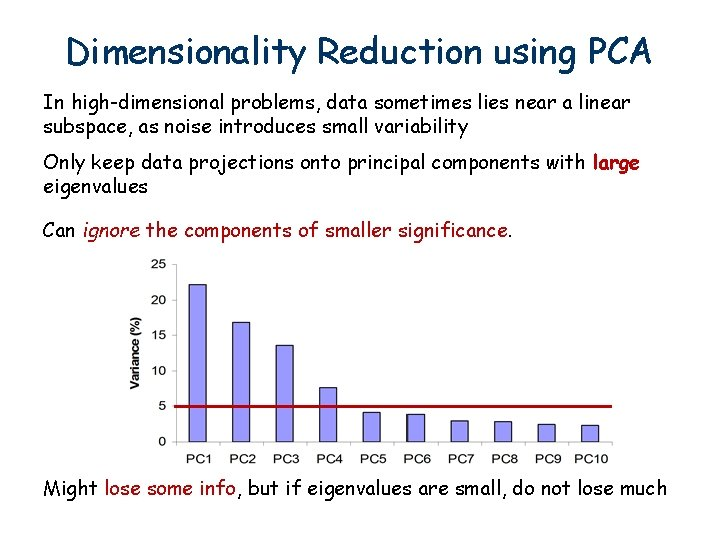Dimensionality Reduction using PCA In high-dimensional problems, data sometimes lies near a linear subspace, as noise introduces small variability Only keep data projections onto principal components with large eigenvalues Can ignore the components of smaller significance. Might lose some info, but if eigenvalues are small, do not lose muchCan represent a face image using just 15 numbers!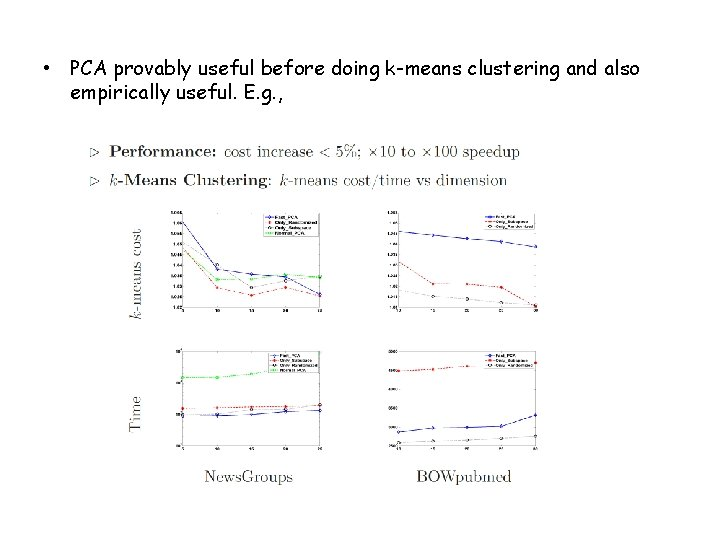• PCA provably useful before doing k-means clustering and also empirically useful. E. g. ,PCA Discussion Strengths Eigenvector method No tuning of the parameters No local optima Weaknesses Limited to second order statistics Limited to linear projections 21What You Should Know • Principal Component Analysis (PCA) • What PCA is, what is useful for. • Both the maximum variance subspace and the minimum reconstruction error viewpoint.